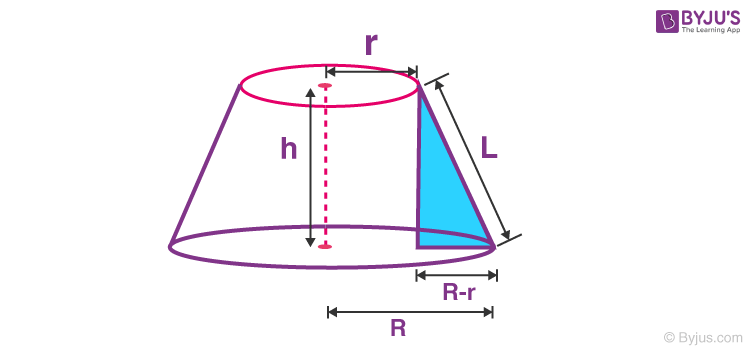# Frustum of a Right Circular Cone Formula

Frustum of a right circular cone is that portion of right circular cone included between the base and a section parallel to the base not passing through the vertex.Properties of Frustum of Right Circular Cone

• The altitude of a frustum of a right circular cone is the perpendicular distance between the two bases. It is denoted by h.
• All elements of a frustum of a right circular cone are similar to a cone. Here, slant height is denoted by L.
 The formula of Frustum of a Right Circular Cone CSA = πL(R + r) TSA = πL(R + r) + πR2+ πr2 V0lume, V = $$\begin{array}{l}\frac{\pi h}{3} \left ( R^{2}+ Rr + r^{2} \right)\end{array}$$

### Solved Example

Question: Find the volume of a frustum of a right circular cone with height 20, lower base radius 34 and top radius 19.

Solution:

Given,
h = 20
R = 34
r = 19

V =

$$\begin{array}{l}\frac{\pi h}{3} \left ( R^{2}+ Rr + r^{2} \right)\end{array}$$

V =

$$\begin{array}{l}\frac{\pi \times 20}{3} \left (34^{2} + 34 \times 19 + 19^{2} \right)\end{array}$$

V = 14420 π cubic units

#### 1 Comment

1. Roshni

Absolutely loved the way we’re addressed as soon as possible!! The service is so good . Here for the first time and I received such a good response. Definitely love this app!💛